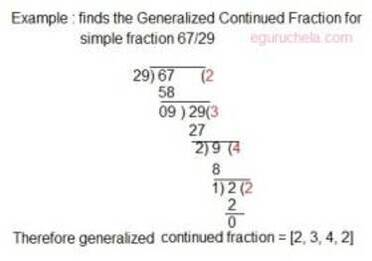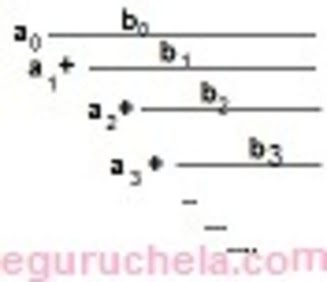# Continued Fractions Calculation

Calculator finds the Generalized Continued Fraction for given simple fraction.

 Enter a simple fraction :

 Generalized Continued Fraction

It is an infinite expression obtained for entered fraction by writing a number as a sum of its integer part and the reciprocal of another number and again the sum of integer of the other number and another reciprocal and so on.

The continued fractions are useful in finding rational approximations to irrational numbers.

## Example### What is the continued fraction

The continued fraction refers to all expressions of the form as follows:where a1,a2,a3,.... and b1,b2,b3,... are either real or complex values. The number of terms can be either finite or infinite.